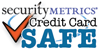item# 2135-2000

# 30" Clear Round Neck Tube Lanyard with reattachable post connector

SIZE
30" long ( metric: 762mm )
THICKNESS
approximately 1/8" diameter
QUANTITY
each
MATERIAL
vinyl
CONNECTOR post
COLOR
Clear

Quantity:

QUANTITY DISCOUNT PRICING

1 to 9 = \$1.45 / each

10 to 24 = \$1.19 / each

25 to 49 = \$0.67 / each

50 to 99 = \$0.53 / each

100 to 249 = \$0.35 / each

250 to 499 = \$0.30 / each

500 to 999 = \$0.27 / each

1000 to 2499 = \$0.26 / each

2500 to 9999 = \$0.25 / each